# GSEB Solutions Class 11 Physics Chapter 12 Thermodynamics

Gujarat Board GSEB Textbook Solutions Class 11 Physics Chapter 12 Thermodynamics Textbook Questions and Answers.

## Gujarat Board Textbook Solutions Class 11 Physics Chapter 12 Thermodynamics

### GSEB Class 11 Physics Thermodynamics Text Book Questions and Answers

Question 1.
A geyser heats water flowing at the rate of 3.0 litres per minute from 27°C to 77°C. If the geyser operates on a gas burner, what is the rate of consumption of fuel if its heat of consumption is 4.0 × 104 J/g?
Here volume of water heated,
V = 3.0 litre min-1
= 3 × 103m-3 min-1
ρ of water = 103 kg m-3
∴ mass of water heated, m = ρV
= 103 × 3 × 10-3kg min-1
= 3 kg min-1
Rise in temperature of water,
∆θ = θ2 – θ1 = 77 – 27 = 50°C
Specific heat of water, C = 4.2 J g-1C-1
= 4.2 × 103 J g-1C-1
Heat required by water = ∆θ = mc∆θ
= 3 4.2 × 103 × 50 J min-1
= 63 × 104 J min-1 …………………… (1)
Heat of combustion of fuel = 4.0 × 104 J g-1 = 4.0 × 107 J kg-1
Let m kg min-1 be the rate of combustion of fuel.
∴ Heat supplied by gas burner of combustion of fuel
= m × 4 × 107 J/min ……………………. (2)
∴ From (1) and (2), we get
63 × 104 = m × 4 × 107
or m = $$\frac{63 \times 10^{4}}{4 \times 10^{7}}$$
= 15.75 × 10-3 kg min-1.
or rate of combustion of fuel = 16 g min-1.Question 2.
What amount of heat must be supplied to 2.0 × 10-2 kg of nitrogen (at room temperature) to raise its temperature by 45°C at constant pressure? (Molecular mass of N2 = 28, R = 8.3 J mol-1K-1).
Here, mass of gas, m = 2 × 10-2 kg = 20 g
Rise in temperature, ∆T = 45°C
Molar mass of N2, M = 28 gm
If n = no. of moles in m, then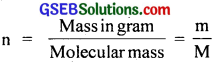= $$\frac{20}{28}$$ = 0.714
R = 8.3 J mol-1K
If Cp be the molar specific heat of the gas at constant pressure, then
Cp = $$\frac{7}{2}$$R = $$\frac{7}{2}$$ × 8.3 Jmol-1
Heat supplied, Q = ?
Using the relation, Q = nCp∆θ, we get
Q = nCp∆T
= 0.714 × $$\frac{7}{2}$$ × 8.3 × 45 J
= 933.75 J = 934 J.

Question 3.
Explain why:
(a) Two bodies at different temperatures T1 and T2 if brought in thermal contact don’t necessarily settle to the mean temperature (T1 + T2)/2.
(b) The coolant in a chemical on a nuclear plant (i.e, liquid used to prevent the different parts of a plant from getting, too hot) should have high specific heat.
(c) Air pressure in a car tyre increases during driving.
(d) The climate of a harbour town is more temperate than that of a town in a desert at the same latitude.
(a) This is because the two bodies may have different masses and different specific heats. In thermal contacts, heat flows from the body at higher temperature to the body at lower temperature till temperature of the two become equal. The final temperature can be the mean temperature i. e. $$\frac{\mathrm{T}_{1}+\mathrm{T}_{2}}{2}$$ only when the thermal capacities of the two bodies are equal.

(b) The function of the coolant in a chemical or nuclear plant is to absorb the heat produced and hence to prevent its different parts from getting too hot. It has high specific heat. The heat absorbed by a substance is directly proportional to the specific heat of the substance. Thus higher the value of specific heat, more will be the heat absorbed by the coolant for the same rise of temperature and hence the plant is protected from overheating.

(c) The temperature of tyre and hence of the air inside it increases during driving, due to friction between the tyres and the road. Now the volume of the tyre remains unchanged practically. So according to Charle’s law (P ∝ T), due to increase in temperature, the air pressure inside the tyre increases.

(d) This is due to the presence of a large amount of water vapours in air due to the proximity with sea.Question 4.
A cylinder with a movable piston contains 3 moles of hydrogen at standard temperature and pressure. The walls of the cylinder are made Of heat insulator, and the piston is insulated by having a pile of sand on it. By what factor does the pressure of the gas increase if the gas is compressed to half its original volume?
Here, let V1 = x
V2 = $$\frac{V_{1}}{2}$$ = $$\frac{x}{2}$$
γ = $$\frac{\mathrm{C}_{\mathrm{p}}}{\mathrm{C}_{\mathrm{v}}}$$
for diatomic gas or hyudrogen,
γ = $$\frac{7}{5}$$ = 1.4
$$\frac{P_{2}}{P_{1}}$$ = ?
As no heat is allowed to be exchanged, the process is adiabatic. Now for adiabatic change,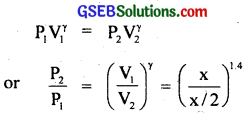= (2)14 = 2.64.

Question 5.
In changing the state of a gas adiabatically from an equilibrium state A to another equilibrium state B, an amount of work equal to 22.3 J is done on the system. If the gas is taken from state A to B via a process in which the net heat absorbed by the system is 9.35 cal, how much is the net work done by the system in the latter case? (Take 1 cal = 4.19 J)?
Here, when the state of the gas changes adiabatically from A to B, the amount of work done is used to increase the internal energy of the gas.
As dW = work done = 22.3 J
If dU = increase in internal energy, then
dU = dW = 22.3 J ……………… (i)
In second case, when the state A is taken to state B, the heat absorbed by the system ∆Q is:
∆Q = 9.35 cal
= 9.35 × 4.19 J (∵ 1 cal = 4.19 J)
= 39.18 J
Let ∆W’ be the net work done by the system, then using first law of thermodynamics,
∆Q = ∆U + ∆W, we get
∆W’ = ∆Q – ∆U
= 39.18 – 22.3
= 16.88 J
= 16.9 J.Question 6.
Two cylinders A and B of equal capacity are connected to each other via a stopcock. A contains a gas at standard temperature and pressure. B is completely evacuated. The entire system is thermally insulated. The stopcock is suddenly opened?
(a) What is finally pressure of the gas in A and B?
(b) What is the change in internal energy of the gas?
(c) What is the change in temperature of the gas?
(d) Do the intermediate states of the system (before settling to the final equilibrium state) lie on its P-V-T surface?’
(a) As the initial and final temperature remain the same. When the stopcock is suddenly opened, the volume available to the gas at 1 atmosphere pressure will become two times. Thus pressure will decrease to one-half i.e. 0.5 atmosphere.
i.e. P2V2 = P1V1
Here, P1 = 1 atm
V1 = V
∴ V2 = 2v
P2 = ?
∴ P2 = $$\frac{P_{1} V_{1}}{V_{2}}$$
= $$\frac{1×V}{2v}$$
= 0.5 atm.

(b) Zero. This is because no work is done on/by the gas, thus there will be no change in the internal energy of the gas under constant temperature conditions.

(c) If the gas is assumed to be ideal, then there is no change in the temperature of the gas as it does no work in expansion i.e. ∆T = 0.

(d) No, since the process (called free expansion) is rapid and cannot be controlled. The intermediate states of the system (gas here) are non-equilibrium states and don’t satisfy the gas equation so it does not lie on the P-V-T surface. In due course, the gas does return to an equilibrium state which lies on its P-V-T surface.Question 7.
A steam engine delivers 5.4 × 108 J of work per minute and services 3.6 × 109 J of heat per minute from the boiler. What is the efficiency of the engine? How much heat is wasted per minute?
IIere, Q1 = heat absorbed from the boiler per minute = 3.6 × 109
W = work done per minute by the steam engine = 5.4 × 108 J
Q2 = heat wasted / rejected per minute = ?
η% = percentage efficiency of the engine = ?
We know that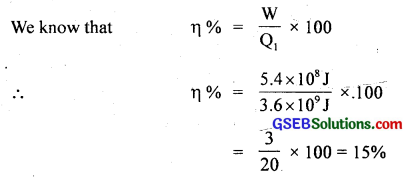Also using the relation, Q1 = W + Q2, we get
Q2 = Q1 – W
= 36 × 108 – 5.4 × 108
= 30.6 × 108 J/min
= 3.06 × 109 J/min
= 3.1 × 109 J/min

Question 8.
An electric heater supplies heat to a system at a rate of 100 W. If the system performs workata rate of 75 joules per second. At what rate is the internal energy increasing?
Here, heat supplied per second, dQ = 100 J
Work done by the system per second, dW = 75 J
Increase in internal energy/second dU = ?
According to first law of thermodynamics, we know that
dQ = dU + dW
∴ dU = dQ – dW
= 100 J – 75 J
= 25 Jper second
= 25 W (∵ 1 watt = 1 Js-1)
∴ dU = 25W.Question 9.
A thermodynamic system is taken from an original state to an intermediate state by linear process shown in figure here. Its volume is then reduced to the original value from E to F by an isobaric process. Calculate the total work done by the gas from D to E to F?
Let W = total work done by the gas from D to E to F.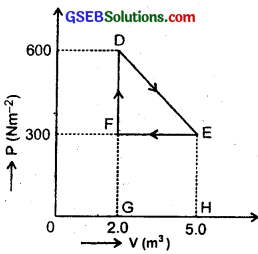If W1 and W2 be the work done by the gas from D to E and E to F respectively, then
W = W1 + W2 …………………… (1)
Now W1 = work done during the process from D to E (expansion) = area DEHGD = area of ADEF + area of rectangle EHGF
= $$\frac{1}{2}$$EF × DF + GH × FG …………………….. (2)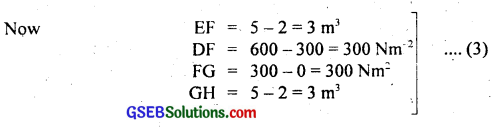∴ From (2) and (3), we get
∴ W1 = [$$\frac{1}{2}$$ × 3 × 300 + 3 × 300]J ……………………… (4)
W2 = work done during the process from E to F (compression)
= Area EHGF
= – FG × GH
= – (300 – 0) × (5 – 2)
= – 300 × 3 J ………………………….. (5)
∴ From (1), (4) and (5), we get
W = $$\frac{1}{2}$$ × 3 × 300 + 3 × 300 – 300 × 3
= 3 × 150 J
= 450 J.Question 10.
A refrigerator is to remove heat from the eatable kept inside at 9°C. Calculate the coefficient of performance if the room temperature is 36°C?
Using the relation, β = $$\frac{\mathrm{T}_{2}}{\mathrm{~T}_{1}-\mathrm{T}_{2}}$$, we get
β = $$\frac{282}{309-282}$$ = $$\frac{282}{27}$$